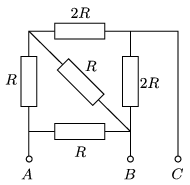Mathematical and Physical Journal
for High Schools
Issued by the MATFUND Foundation
 Already signed up? New to KöMaL?

#Problem P. 4968. (October 2017)

P. 4968. The heating elements of an electric heater can be connected as shown in the figure. When voltage is applied across points $\displaystyle A$ and $\displaystyle B$, then during a certain amount of time, water of mass $\displaystyle m$ can be boiled. How much water can be boiled during the same amount of time when the voltage is applied across points $\displaystyle B$ and $\displaystyle C$ or across points $\displaystyle C$ and $\displaystyle A$?(4 pont)

Deadline expired on November 10, 2017.

Sorry, the solution is available only in Hungarian. Google translation

Megoldás. Az egyes pontpárok közötti eredő ellenállás:

$\displaystyle R_{AB}=\frac9{14}R,\qquad R_{BC}=\frac87\,R,\qquad R_{AC}=\frac32 R.$

Adott feszültség mellett a leadott teljesítmény, és ezzel együtt a felforralható víz mennyisége az eredő ellenállásokkal fordítottan arányos:

$\displaystyle m_{AB}=m,\qquad m_{BC}=\frac9{16}\,m\approx0{,}56\,m ,\qquad m_{AC}=\frac{3}{7}\,m\approx0{,}43\,m.$

### Statistics:

 83 students sent a solution. 4 points: 54 students. 3 points: 20 students. 2 points: 5 students. 1 point: 2 students. 0 point: 2 students.

Problems in Physics of KöMaL, October 2017